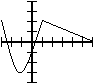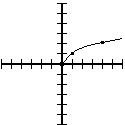# 1.9 Graphing  (Page 3/3)

 Page 3 / 3

## Other vertical permutations

Adding or subtracting a constant from $f\left(x\right)$ , as described above, is one example of a vertical permutation: it moves the graph up and down. There are other examples of vertical permutations.

For instance, what does doubling a function do to a graph? Let’s return to our original function:y = f ( x ) size 12{y=f $$x$$ } {}

What does the graph $y=2f\left(x\right)$ look like? We can make a table similar to the one we made before.

$x$ $f\left(x\right)$ $2f\left(x\right)$ so $y=2f\left(x\right)$ contains this point
–3 2 4 $\left(-3,4\right)$
–1 –3 –6 $\left(-1,-6\right)$
1 2 4 $\left(1,4\right)$
6 0 0 $\left(6,0\right)$

In general, the high points move higher; the low points move lower. The entire graph is vertically stretched , with each point moving farther away from the x-axis.y = f ( x ) size 12{y=f $$x$$ } {}

Similarly, $y=\frac{1}{2}f\left(x\right)$ yields a graph that is vertically compressed, with each point moving toward the x-axis.

Finally, what does $y=-f\left(x\right)$ look like? All the positive values become negative, and the negative values become positive. So, point by point, the entire graph flips over the x-axis.y = f ( x ) size 12{y=f $$x$$ } {}

## What happens to the graph, when you add 2 to the x value?

Vertical permutations affect the y-value; that is, the output, or the function itself. Horizontal permutations affect the x-value; that is, the numbers that come in. They often do the opposite of what it naturally seems they should.

Let’s return to our original function $y=f\left(x\right)$ .y = f ( x ) size 12{y=f $$x$$ } {} ; Contains the following points (among others): ( − 3,2 ) size 12{ $$- 3,2$$ } {} , ( − 1, − 3 ) size 12{ $$- 1, - 3$$ } {} , ( 1,2 ) size 12{ $$1,2$$ } {} , ( 6,0 ) size 12{ $$6,0$$ } {}

Suppose you were asked to graph $y=f\left(x+2\right)$ . Note that this is not the same as $f\left(x\right)+2$ ! The latter is an instruction to run the function, and then add 2 to all results. But $y=f\left(x+2\right)$ is an instruction to add 2 to every x-value before plugging it into the function.

• $f\left(x\right)+2$ changes $y$ , and therefore shifts the graph vertically
• $f\left(x+2\right)$ changes $x$ , and therefore shifts the graph horizontally.

But which way? In analogy to the vertical permutations, you might expect that adding two would shift the graph to the right. But let’s make a table of values again.

$x$ $x+2$ $f\left(x+2\right)$ so $y=f\left(x+2\right)$ contains this point
–5 –3 f(–3)=2 $\left(-5,2\right)$
–3 –1 f(–1)=–3 $\left(-3,-3\right)$
–1 1 f(1)=2 $\left(-1,2\right)$
4 6 f(6)=0 $\left(4,0\right)$

This is a very subtle, very important point—please follow it closely and carefully! First of all, make sure you understand where all the numbers in that table came from. Then look what happened to the original graph.

The original graph $f\left(x\right)$ contains the point $\left(6,0\right)$ ; therefore, $f\left(x+2\right)$ contains the point $\left(4,0\right)$ . The point has moved two spaces to the left.y = f ( x ) size 12{y=f $$x$$ } {}

You see what I mean when I say horizontal permutations “often do the opposite of what it naturally seems they should”? Adding two moves the graph to the left .

Why does it work that way? Here is my favorite way of thinking about it. $f\left(x-2\right)$ is an instruction that says to each point, “look two spaces to your left, and copy what the original function is doing there .” At $x=5$ it does what $f\left(x\right)$ does at $x=3$ . At $x=\text{10}$ , it copies $f\left(8\right)$ . And so on. Because it is always copying $f\left(x\right)$ to its left , this graph ends up being a copy of $f\left(x\right)$ moved to the right . If you understand this way of looking at it, all the rest of the horizontal permutations will make sense.

Of course, as you might expect, subtraction has the opposite effect: $f\left(x-6\right)$ takes the original graph and moves it 6 units to the right . In either case, these horizontal permutations affect the domain of the original function, but not its range .

## Other horizontal permutations

Recall that $y=2f\left(x\right)$ vertically stretches a graph; $y=\frac{1}{2}f\left(x\right)$ vertically compresses . Just as with addition and subtraction, we will find that the horizontal equivalents work backward.

$x$ $2x$ $f\left(2x\right)$ so $y=2f\left(x\right)$ contains this point
–1½ –3 2 $\left(-1\frac{1}{2},2\right)$
–½ –1 –3 $\left(-\frac{1}{2};-3\right)$
½ 1 2 $\left(\frac{1}{2};2\right)$
3 6 0 $\left(3,0\right)$

The original graph $f\left(x\right)$ contains the point $\left(6,0\right)$ ; therefore, $f\left(2x\right)$ contains the point $\left(3,0\right)$ . Similarly, $\left(-1;-3\right)$ becomes $\left(-\frac{1}{2};-3\right)$ . Each point is closer to the y-axis; the graph has horizontally compressed .y = f ( x ) size 12{y=f $$x$$ } {}

We can explain this the same way we explained $f\left(x-2\right)$ . In this case, $f\left(2x\right)$ is an instruction that says to each point, “Look outward, at the x-value that is double yours, and copy what the original function is doing there .” At $x=5$ it does what $f\left(x\right)$ does at $x=\text{10}$ . At $x=-3$ , it copies $f\left(-6\right)$ . And so on. Because it is always copying f(x) outside itself, this graph ends up being a copy of $f\left(x\right)$ moved inward ; ie a compression. Similarly, $f\left(\frac{1}{2}x\right)$ causes each point to look inward toward the y-axis, so it winds up being a horizontally stretched version of the original.

Finally, $y=f\left(-x\right)$ does precisely what you would expect: it flips the graph around the y-axis. $f\left(-2\right)$ is the old $f\left(2\right)$ and vice-versa.y = f ( x ) size 12{y=f $$x$$ } {}

All of these permutations do not need to be memorized: only the general principles need to be understood. But once they are properly understood, even a complex graph such as $y=-2\left(x+3{\right)}^{2}+5$ can be easily graphed. You take the (known) graph of $y={x}^{2}$ , flip it over the x-axis (because of the negative sign), stretch it vertically (the 2), move it to the left by 3, and move it up 5.

With a good understanding of permutations, and a very simple list of known graphs, it becomes possible to graph a wide variety of important functions. To complete our look at permutations, let’s return to the graph of $y=\sqrt{x}$ in a variety of flavors.y = x size 12{y= sqrt {x} } {} ; Generated by plotting points; Contains ( 0,0 ) size 12{ $$0,0$$ } {} , ( 1,1 ) size 12{ $$1,1$$ } {} , ( 4,2 ) size 12{ $$4,2$$ } {} ; Domain: x ≥ 0 size 12{x>= 0} {} ; Range: y ≥ 0 size 12{y>= 0} {} ; Range: y ≥ 0 size 12{y>= 0} {}

who are u?
Lamine
haha
Cleaford
scarm
nura
what it this
Cleaford
hi y'all
Dope
how does group chat help y'all 🤔
Dope
hi y'all
Dope
how does group chat help y'all 🤔
Dope
how does group chat help y'all 🤔
Dope
to learn from one another
Lamine
oh okay
Dope
😟
Creative
Yes
Lamine
what is type of economic
how to understand basics of economics
what is demand schedle
When you make a Scedule of the demand you made
Rodeen
What is macroeconomics
It's one of the two branches of Economics that deal with the aggregate economy.
Mayen
it's about inflation, occupation, gdp and so on
alberto
What is differences between Microeconomics and Macroeconomic?
Bethrand
a price floor of 24 imposed
monopolistic competition
yap
nura
any one there to answer my question
Fixed Costs per week Variable Costs per bear Rent & Rates of Factory Hire & machines Heating & Lighting Repayment of Bank Loan K100.00 K45.00 K5.00 K50.00 Materials Foam Wages K6.00 K1.00 K1.00 Total K200.00 K8.00
Richard
one of the scarce resources that constrain our behaviour is time. each of us has only 24 hours in a day. how do you go about allocating your time in a given day among completing alternatives? once you choose a most important use of time. why do you not spend all your time to it. use the notion of op
mohsina mala..Bangla app hobe na
mani Baba. First learn the spelling of Economics
Economics- The study of how people use their limited resources to tey and satisfy unlimited wants.
Kelly
hmmm
Mani
etar bangla apps hobe na?
Mohsina
what is defination of acnomics
বাংলা বই পাওয়া যায় না?
what is a commdity
in a comparison of the stages of meiosis to the stage of mitosis, which stages are unique to meiosis and which stages have the same event in botg meiosis and mitosis
Got questions? Join the online conversation and get instant answers!By Keyaira BraxtonBy OpenStaxBy Rohini AjayBy OpenStaxBy Edward BitonBy OpenStaxBy Dravida Mahadeo-J...By Brooke DelaneyByBy OpenStax